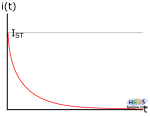What happens when our bottle is full and source excluded?

Imagine circuit in first page now without V, substituted by a line directed from R to Ground (the strange symbol that seems a bottom-up tower).

Remember C's voltage value?
That's V, with a starting I$_{ST}\not=$0.

So now R sees V at its right and zero-reference (Ground: G) on left: C pulls charges to G through R until its voltage differs from reference value.

See current behavior on above pictures.

Time requested? Sure! always$R\cdot C$ to reach$\frac{2}{3}$ of its maximum, and$5R\cdot C$ to get zero.

Quick quiz: What if we cancel V source without adding a line, leaving circuit open? Will C discharge anyway?

Yes.

Joule (energy: J) effect is present;  C slowly looses its accumulated energy ($Q\cdot V$ gives J, i.e. Coulomb$\cdot$Volt = Energy).

Joule effect concerns  power dissipation by electrical component when submitted to a flowing current; this means that the first includes the last and vice-versa.

This happens in previous case too, because heat exchange is a must in nature.

Here below two pictures of circuit without power supply (second situation)

Difference between them is red LED on in first and off in second; talking about software everything will be clear.